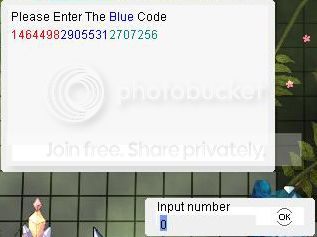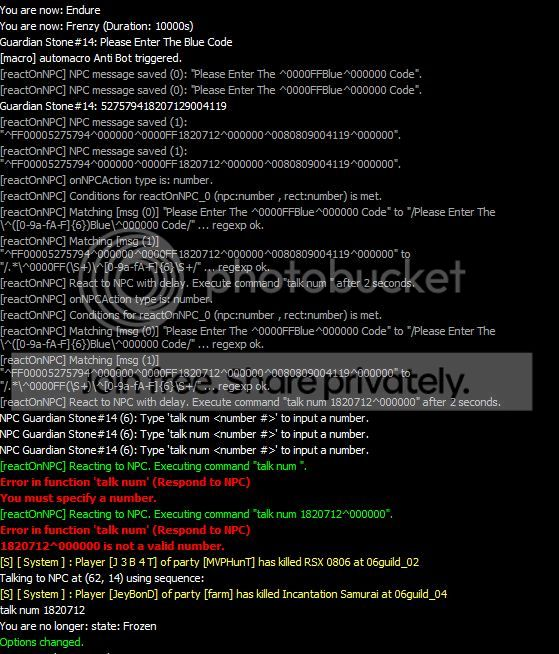Page 44 of 44

### Re: reactOnNPC v.2.0.2 by hakore

Posted: 20 Nov 2018, 14:30
c4c1n6kr3m1 wrote:
headlesscarmen wrote: anyone has a solution, for 3 days im dreading this.haha
can you test this style? this one accept plus minus sign or others math operator

Code: Select all

``````reactOnNPC_debug 1
reactOnNPC eval {my \$number1 = "#1~1";my \$number2 = "#1~1";my \$opsign = "#2~1";my \$answer = eval("\$number1 \$opsign \$number2");message("The answer is \$answer\n","info");Commands::run("talk num \$anwser")} {
type number
useColors 1
delay 5
msg_0 /\[ Anti BOT System \]/
msg_1 /If A = (\d+)\s+B = (\d+)/
msg_2 /How many is A (.) B ?/
}
``````
Hi,thanks for the god's work u doing here answering question.

here is the debug code after i tested it.
You are now: state: Frozen
Options changed.
You are now immune to attacks.
You are now: Endure
You are now: Berserk (Duration: 1000000s)
Unknown #110014448: [ Anti BOT System ]
[reactOnNPC] NPC message saved (0): "[ Anti BOT System ]".
Unknown #110014448: If A = 67 B = 5
[reactOnNPC] NPC message saved (1): "If A = 67 B = 5".
Unknown #110014448: How many is A - B ?
[reactOnNPC] NPC message saved (2): "How many is A - B ?".
[reactOnNPC] onNPCAction type is: number.
[reactOnNPC] Conditions for reactOnNPC_0 (npc:number , rect:number) is met.
[reactOnNPC] Matching [msg (0)] "[ Anti BOT System ]" to "/\[ Anti BOT System \]/" ... regexp ok.
[reactOnNPC] Matching [msg (1)] "If A = 67 B = 5" to "/If A = (\d+)\s+B = (\d+)/" ... regexp ok.
[reactOnNPC] Matching [msg (2)] "How many is A - B ?" to "/How many is A (.) B ?/" ... regexp ok.
[reactOnNPC] React to NPC with delay. Execute command "eval {my \$number1 = "67";my \$number2 = "67";my \$opsign = "-";my \$answer = eval("\$number1 \$opsign \$number2");message("The answer is \$answer\n","info");Commands::run("talk num \$anwser")}" after 5 seconds.
: Type 'talk num <number #>' to input a number.
Allowed other player invite to Party
Other players are not allowed to view your Equipment.
[reactOnNPC] Reacting to NPC. Executing command "eval {my \$number1 = "67";my \$number2 = "67";my \$opsign = "-";my \$answer = eval("\$number1 \$opsign \$number2");message("The answer is \$answer\n","info");Commands::run("talk num \$anwser")}".
Syntax Error in function 'talk' (Talk to NPC)
Usage: talk <NPC # | "NPC name" | cont | resp | num | text > [<response #>|<number #>]

this work very well but only for addition question. The anti bot system ask addition and subtraction question.

### Re: reactOnNPC v.2.0.2 by hakore

Posted: 21 Nov 2018, 04:03
c4c1n6kr3m1 wrote:
headlesscarmen wrote: anyone has a solution, for 3 days im dreading this.haha
can you test this style? this one accept plus minus sign or others math operator

Code: Select all

``````reactOnNPC_debug 1
reactOnNPC eval {my \$number1 = "#1~1";my \$number2 = "#1~1";my \$opsign = "#2~1";my \$answer = eval("\$number1 \$opsign \$number2");message("The answer is \$answer\n","info");Commands::run("talk num \$anwser")} {
type number
useColors 1
delay 5
msg_0 /\[ Anti BOT System \]/
msg_1 /If A = (\d+)\s+B = (\d+)/
msg_2 /How many is A (.) B ?/
}
``````
Hi,thanks for the god's work u doing here answering question.

here is the debug code after i tested it.
You are now: state: Frozen
Options changed.
You are now immune to attacks.
You are now: Endure
You are now: Berserk (Duration: 1000000s)
Unknown #110014448: [ Anti BOT System ]
[reactOnNPC] NPC message saved (0): "[ Anti BOT System ]".
Unknown #110014448: If A = 67 B = 5
[reactOnNPC] NPC message saved (1): "If A = 67 B = 5".
Unknown #110014448: How many is A - B ?
[reactOnNPC] NPC message saved (2): "How many is A - B ?".
[reactOnNPC] onNPCAction type is: number.
[reactOnNPC] Conditions for reactOnNPC_0 (npc:number , rect:number) is met.
[reactOnNPC] Matching [msg (0)] "[ Anti BOT System ]" to "/\[ Anti BOT System \]/" ... regexp ok.
[reactOnNPC] Matching [msg (1)] "If A = 67 B = 5" to "/If A = (\d+)\s+B = (\d+)/" ... regexp ok.
[reactOnNPC] Matching [msg (2)] "How many is A - B ?" to "/How many is A (.) B ?/" ... regexp ok.
[reactOnNPC] React to NPC with delay. Execute command "eval {my \$number1 = "67";my \$number2 = "67";my \$opsign = "-";my \$answer = eval("\$number1 \$opsign \$number2");message("The answer is \$answer\n","info");Commands::run("talk num \$anwser")}" after 5 seconds.
: Type 'talk num <number #>' to input a number.
Allowed other player invite to Party
Other players are not allowed to view your Equipment.
[reactOnNPC] Reacting to NPC. Executing command "eval {my \$number1 = "67";my \$number2 = "67";my \$opsign = "-";my \$answer = eval("\$number1 \$opsign \$number2");message("The answer is \$answer\n","info");Commands::run("talk num \$anwser")}".
Syntax Error in function 'talk' (Talk to NPC)
Usage: talk <NPC # | "NPC name" | cont | resp | num | text > [<response #>|<number #>]

this work very well but only for addition question. The anti bot system ask addition and subtraction question.
ah sorry i made mistake, it's because 67 - 67 = 0

\$number2 should "#1~2"
try this

Code: Select all

``````reactOnNPC eval {my \$number1 = "#1~1";my \$number2 = "#1~2";my \$opsign = "#2~1";my \$answer = eval("\$number1 \$opsign \$number2");message("The answer is \$answer\n","info");Commands::run("talk num \$answer")} {
``````

Code: Select all

``````reactOnNPC eval {my \$number1 = "#1~1";my \$number2 = "#1~2";my \$opsign = "#2~1";my \$answer = eval("\$number1 \$opsign \$number2");message("The answer is \$answer\n","info");\$messageSender->sendTalkNumber(\$talk{'ID'}, \$answer)} {
``````

### Re: reactOnNPC v.2.0.2 by hakore

Posted: 06 Apr 2019, 02:10

[reactOnNPC] NPC message saved (0): "[ 反外挂剩余 ^ff00003^000000 次验证机会]".

[reactOnNPC] NPC message saved (1): "- 您还剩余 ^ff00001^000000分^ff000030^000000秒验证时间。".

[reactOnNPC] NPC message saved (2): " 防止挂机,请用数字完成验证.".

[reactOnNPC] NPC message saved (3): " ^e5387d７^000000 + ^0066FF１０^000000 〓 （ ? ）".

[reactOnNPC] onNPCAction type is: number.

reactOnNPC_debug 1
reactOnNPC eval {my \$number1 = "#1~1";my \$number2 = "#1~3";my \$opsign = "#1~2";my \$answer = eval("\$number1 \$opsign \$number2");message("The answer is \$answer\n","info");Commands::run("talk num \$answer")} {
type number
useColors 1
delay 5
msg_0 / 防止挂机,请用数字完成验证./
msg_1 /\^[0-9a-fA-F]{6}(\d+)\^000000\s(.)\s\^[0-9a-fA-F]{6}(\d+)\^000000/

}Numbers are special characters,Can not work
help me

### Re: reactOnNPC v.2.0.2 by hakore

Posted: 08 Sep 2019, 03:47
anyone know why my npc react with "^000000" at the end? .. i dont know how to solve this..i'm using this right now :

Code: Select all

``````reactOnNPC_debug 1
reactOnNPC talk num @eval(my \$color1 = '#0~0';my \$color2 = '#1~0';if (\$color1 eq \$color2@) {return '#1~1'}) {
type number
useColors 1
delay 2
msg_0 /Please Enter The \^([0-9a-fA-F]{6})Blue\^000000 Code/
msg_1 /.*\^0000FF(\S+)\^[0-9a-fA-F]{6}\S+\^000000/
}``````